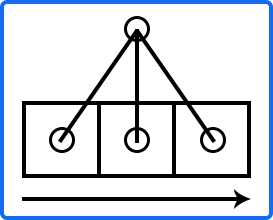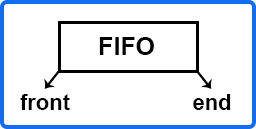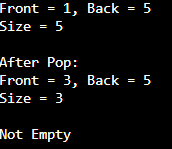# Queue in C++

## Queue in C++ with Examples:

In this article, I am going to discuss Queue in C++ with Examples. Please read our previous article, where we discussed Stack in C++ with Examples. In this article, we will look at another container adapter which is the queue. It follows the first in first out principle which is FIFO.

##### Queue in C++ with Examples:

In Stack, we had seen that stack follows last in first out. So whatever element we put in the end will be the one that will be popped first. Here, it is the opposite. It is like you are in a queue for something or maybe a movie ticket and the person who came first or is the first in the queue will get processed first. So that person will get out of the queue first then next and so on. These data structures are used a lot in multiple problems.

For example, let us say you are doing a breadth-first Search of the graph. So, you insert all the neighbors of a node in a queue. These are in the queue so these will be processed from left to right,Then first you will pop the left element then you will push its neighbors. These are pushed in the end. So, these will be processed level by level. This is how we implement breadth-first search. There are many more applications in the queue.

These are said to be container adapters and the by default underlying container will be deque. So deque has both push and pop functions from both the front and back of the end. The class template act as a wrapper to the underlying container i.e. deque. And it exposes only the functionalities that are required in order to implement a queue data structure. In the queue, you can only push in the end and pop from the front. That is FIFO.In the case of the deque, it was front and back. Here also, it is front and end. In the queue, you will pop from the front and insert from the back that is we are doing a push operation.

##### Methods of Queue Container in C++

Let us look at some of the operations which are very similar to stack.

1. size() – it will give you the total number of elements present in the queue.
2. = – you can use ‘=’ to assign a new queue. Let’s say you have q1 and you create q2 then you can assign q1 to q2 i.e. q2 = q1.
3. front(), back() – these will give us the front and back elements of the queue.
4. empty() – it will check whether the size is 0 or not.
5. push(), pop() – push will insert the elements from the end and pop will remove the element from the front of the queue.

Now let us look at the program.

##### Example to Understand Queue in C++:
```#include <iostream>
#include <queue>

using namespace std;

int main()
{
// Create a queue of type integer.
queue<int> q;

cout << std::boolalpha << q.empty() << endl; // it will print 1 if queue is empty otherwise 0.
// insert the elements from 1 to 5 in the queue.
for(int i = 1; i <= 5; i++){
q.push(i);
}
// q = {1,2,3,4,5}

cout << "Front = " << q.front() << ", Back = " << q.back() << endl;  // Print the first and last elements of the queue.
cout << "Size = " << q.size() << endl;    // Print the size of the queue.
cout << endl;

// Remove the elements from the queue (from the front).
q.pop();
q.pop();

// q = {3,4,5}
cout << "After Pop: " <<endl;
cout << "Front = " << q.front() << ", Back = " << q.back() << endl;
cout << "Size = " << q.size() << endl;
cout << endl;

if(q.empty())
cout << "Queue is Empty" <<endl;
else
cout << "Not Empty" << endl;

return 0;
}
```
###### Output: